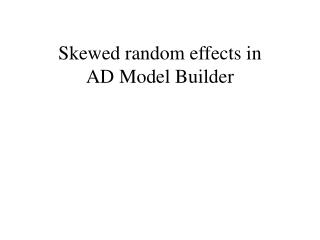DownloadDownload PresentationSkewed random effects in AD Model Builder

# Skewed random effects in AD Model Builder

Download Presentation## Skewed random effects in AD Model Builder

- - - - - - - - - - - - - - - - - - - - - - - - - - - E N D - - - - - - - - - - - - - - - - - - - - - - - - - - -
##### Presentation Transcript

1. Skewed random effects in AD Model Builder

2. Goal • Analyse model in Section 14.2 of Skrondal and Rabe-Hesketh (2004) Generalized Latent Variable Modeling: Multilevel, Longitudinal and Structural Equation Models. Chapman & Hall • Replace normal distribution with a skewed distribution for the random effects.

3. 14.2 Diet and heart disease • Covariate measurement error model • 337 middle aged men (bank, transportation) • At requirement: weigh their food • Repeated measurement for 76 men • Heart disease or not? • Covariates: • age (numeric) • bus (0,1)

4. Model description • Exposure model (true fiber intake) ηj = γ1·agej+γ2·busj + γ3·age*busj+ ζj, ζj ~ N(0, ψ) • Measured fiber intake (i = 1,2) yij = ηj + α0+(i-1)·α1 + ij , ij ~ N(0, θ) • Disease model(D = 0,1) logit(Dj|ηj) = β0 +β1·agej+β2·busj + β3·age*busj + λ·ηj

5. Setting up and running the model • Prepare data in R: ”diet.s” • Compiling the model • admb -re diet • Running the model (Laplace approx.) • diet -est • Running the model (Gauss-Hermite appr.) • diet -est -gh 20

6. Skewed distributions for ζ • Skrondal and Rable-Hesket: • Replace N(0,ψ) distribution for ζj by non-parametric distribution (Fig. 14.2) • Skewed distribution for ζj ζj = [a·uj + (1-a)[exp(uj)-exp(0.5)]/c1]/c2, 0 < a < 1 uj ~ N(0, ψ) c1 = sqrt[e(e-1)], c2 = sqrt(a2 + (1-a)2)